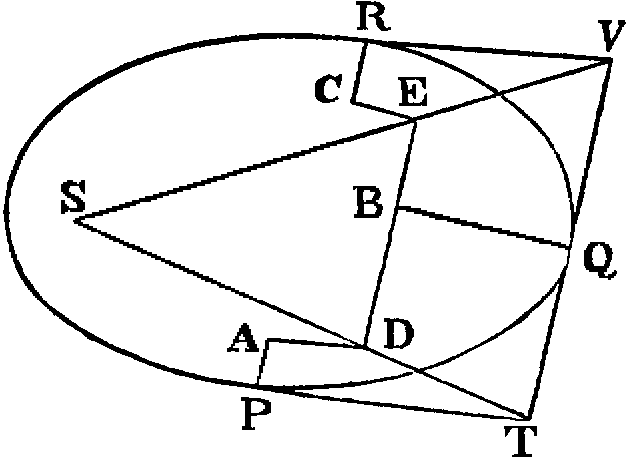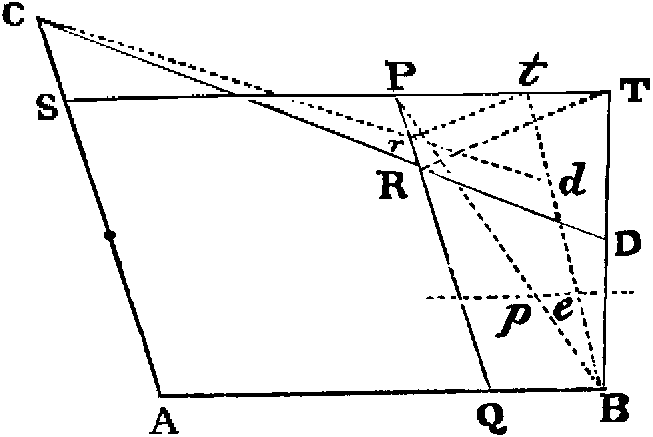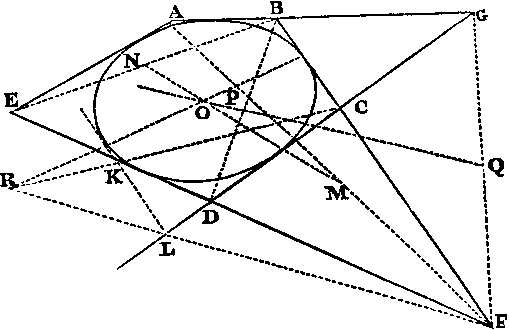# The Pulfrich Effect: Nickalls' Theorem

### PrefaceNickalls' Theorem in geometry first was published in 1986, during a period of active work in vision research. The theorem rigorously may be applied to the Pulfrich effect by assuming the real path of the Pulfrich pendulum to be a conic section in a plane parallel to the floor. Specifically, the path should be assumed to be an ellipse, or, that special case of the ellipse, a circle. The possibility of nulling the illusion to make the apparent path almost a straight line then is derived from the geometry.

Nickalls' theorem links geometry and illusion phenomenology by the assumption that the Pulfrich delay in the filtered eye is a constant function of the illumination difference, under constant experimental conditions--in particular, under constant angular speed of target rotation. This assumption is discussed in papers referenced on the 'Explanation' pages at this web site. Some mathematical context may be found at the 2D-curve site.

R. W. D. Nickalls is an active contributor to this web site. He may be contacted directly by Email.Nickalls' Theorem: Nickalls, R. W. D. Original publication: A line-and-conic theorem having a visual correlate. Mathematical Gazette, 1986, 70, pp. 27 - 29. Nickalls, R. W. D. Supplementary Examples: An explanatory note on Figure 3 of the 1986 derivation. Unpublished manuscript. Nottingham, 1992. Nickalls, R. W. D. New Perspective: A conic theorem generalized: directed angles and applications. The Mathematical Gazette, 2000, 84, pp. 232 - 241. Rigby, J. F. Alternative Proof: A proof of Nickalls' theorem on tangents and foci of a conic. The Mathematical Gazette, 2002, 86, pp. 322 - 324.Other works on the rotating Pulfrich effect by R. W. D. Nickalls are linked externally and include a 1996 paper in Vision Research studying the effect of target angular velocity. This, as well as a 2004 paper in the Swiss Journal of Psychology with S. Nakamizo and H Nawae, have validated Nickalls' Theorem under a variety of stimulus conditions.

The Pulfrich Effect, SIU-C. Last updated 2010-10-15